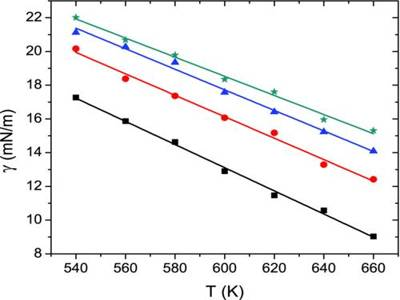When alcohols, phenol, glycerol, fatty acids etc. are dissolved in water, the surface tension of water is reduced, the extent of reduction depending on the concentration of the solute. In such cases, it is noticed that the concentration of the solute is more on the surface layer than in the bulk and the solute is said to be positively adsorbed on the surface. Ionic solids dissolved in a liquid cause the surface tension of the liquid to rise and the concentration of the solute is found to be less on the surface. In such cases, the solute is said to be negatives adsorbed. Gibbs treated these phenomena from thermodynamic principles and denied the following relation:

Γ = – (dγ/dC) (C/RT)

where γ is the surface tension of the solution, Γ the excess concentration of the solute on the surface, C, the concentration of the solute in solution, R, the gas constant and T, the temperature in K. Excess surface concentration (Γ) means the concentration of the solute on the surface in excess of that in the bulk. Consistently all substances that reduce surface tension must have a higher molecular concentration on the surface than in the bulk. As mentioned, electrolytes often have a lower concentration on the surface and they increase the surface tension of water.From a measurement of γ, the surface tension of a series of solutions of different concentrations, C, of a solute, say glycerol, or phenol, a plot of γ vs C is drawn. The gradient of the graph, (dγ/dC), is evaluated at various values of C as shown in Figure. Using the values of the gradient Γ may be calculated with the help of equation. The equation has been experimentally verified by determining Γ by actual chemical analysis of very thin sections of the surfaces of solutions.

The experiment can be carried out in any laboratory because no special or expensive instrument is requital.